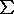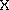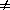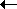Principles of Population Genetics
Various aspects of "Population"

gene pool (a genetic unit):
all the alleles at a (single) locus
deme (an ecological unit):
all the conspecific individuals in an area
panmictic unit (a reproductive unit):
a group of randomly interbreeding individuals
sample (a numerical unit):
a statistical subset of size 'N'
Theory of allele frequencies: p's & q's

Genetic variation in populations can be described by genotype and allele frequencies.
(not "gene" frequencies)

Consider a diploid autosomal locus with two alleles and no dominance
(=> semi-dominance: AA , Aa , aa  phenotypes are distinguishable)

# AA = x    # Aa = y    # aa = z    x + y + z = N (sample size)

f(AA) = x / N       f(Aa) = y / N       f(aa) = z / N

f(A) = (2x + y) / 2N          f(a) = (2z + y) / 2N

or    f(A) = f(AA) + 1/2 f(Aa)      f(a) = f(aa) + 1/2 f(Aa)

let p = f(A), q = f(a)    p & q are allele frequencies

Properties of p & q

p + q = 1     p = 1 - q    q = 1 - p

(p + q)=  p2 + 2pq + q2  =  1

(1 - q)2 + 2(1 - q)(q) + q2 = 1

p & q are interchangeable wrt [read, "with respect to"] A & a;

q is usually used for the
rarer, recessive, or deleterious (disadvantageous) allele;

BUT   'common' & 'rare' are statistical properties
'dominant' & 'recessive' are genotypic properties
'advantageous' & 'deleterious' are phenotypic properties
*** any combination of these properties is possible ***

The Hardy-Weinberg Theorem

What happens to p & q in one generation of random mating?

Consider a population of monoecious organisms reproducing by random union of
gametes ("tide pool" model)...

(1) Determine the expectations
of parental alleles coming together in various genotype combinations.
[expectation: the anticipated value of a variableprobability]
The  probability , binomial expansion , Punnet Square methods
all show that expectation of f(AA) = p2
expectation of f(Aa) = 2pq
expectation of f(aa) = q2

(2) Re-describe allele frequencies among offspring (A' & a').

f(A') = f(AA) + 1/2 f(Aa)
= p2 + (1/2)(2pq) = p2 + pq = p(p+q) = p' = p

f(a') = f(aa) + 1/2 f(Aa)
= q2 + (1/2)(2pq) = q2 + pq = q(p+q) = q' = q

The Hardy - Weinberg Theorem (1908):
In the absence of other genetic or evolutionary factors,
allele frequencies are invariant between generations, and
constant genotype frequencies are reached in one generation.

p2 : 2pq : q2 are Hardy-Weinberg proportions (cf. Mendelian ratios 1 : 2 : 1 )

The Hardy-Weinberg Theorem holds under "more realistic" conditions:

(1) multiple alleles / locus

p + q + r = 1
(p + q + r)2 = p2 + 2pq + q2 + 2qr + r2 + 2pr = 1

The proportion of heterozygotes (H = 'heterozygosity')
is a measure of genetic variation at a locus.

Hobs = f(Aa) = observed heterozygosity
Hexp = 2pq   = expected heterozygosity (for two alleles)

He = 2pq + 2pr + 2qr = 1 - (p2 + q2 + r2)    for three alleles

n
He = 1 -(qi)2      for n alleles
i=1

where qi = freq. of ith allele of n alleles at a locus
Ex.: if q1 = 0.5, q2 = 0.3, & q3 = 0.2
then He = 1 - (0.52 + 0.32 + 0.22) = 0.62

iff [read: "if and only if"] allele frequencies in males and females are identical
If frequencies are initially unequal, they converge over several generations.

(3) dioecious organisms
sexes are separate
H-W is produced by random mating of individuals (random union of genotypes).
expand (p2 'AA' + 2pq 'AB' + q2 'BB')2 :
nine possible 'matings' among genotypes
(See derivation)
No selfing (self-fertilization not possible)

Application of Hardy-Weinberg to population biology

Genotype proportions in natural populations can be tested for H-W conditions
Ho(null hypothesis): no outside factors are acting.

Among North American whites:
 MM MN NN Sum 1787 3039 1303 6129

f(M) = [(2)(1787) + 3039] / (2)(6129)= 0.539

f(N) = [(2)(1303) + 3039] / (2)(6129)= 0.461    = 1.0 - 0.539

Chi-square (2) test:
 # expected observed (obs-exp) d2/exp MM (0.539)2(6129) 1781 1787 6 0.020 MN (2)(0.539)(0.461)(6129) 3046 3039 -7 0.012 NN (0.461)2(6129) 1302 1303 1 0.0002 = 0.032ns
(cf. critical value p.05[1 d.f.] = 3.84)                              ( p >> 0.05)
note: there is only one degree of freedom, because there are only two alleles

But (you ask) won't "expected" always more or less equal "observed",
cuz that's where "expected" comes from?
Consider an artificial data set :

 MM MN NN Sum f(M) f(N) Navajo (US) 305 52 4 361 0.92 0.08 Koori (Aus.) 22 216 492 730 0.18 0.82 Combined 327 268 496 1091 0.42 0.58
(Homework: show that Navajo & Koori populations exhibit H-W proportions)

Chi-square test on combined data:
 exp obs d=(o-e) d2/exp MM 192 327 135 94.9 MN 532 268 -264 131.0 NN 367 496 129 45.32 = 271.2**
(p << 0.01)

*=> A mixture of populations, each of which shows Hardy-Weinberg proportions,
will not show expected Hardy-Weinberg proportions
if the allele frequencies are different in the separate populations.

Wahlund Effect: an artificial mixture of populations will have a deficiency of heterozygotes

Evolutionary Genetics:
modification of Hardy-Weinberg conditions

The Hardy-Weinberg conditions are the 'null hypothesis':
What are the consequences of other genetic / evolutionary phenomena?

Five major factors:

1. Natural selection
Change of allele frequencies (q) [read as 'delta q']
occurs due to differential effects of alleles on 'fitness'
Consequences depend on dominance of fitness (see Lab #1)
Natural Selection is the principle concern of evolutionary theory
(& first half of this course)

2. Mutation
A and A' are inter-converted at some rate µ .
If µ(AA')µ'(AA'), net change will occur in one direction.

3. Gene flow
Net movement of alleles between populations occurs at some rate m .
(Im)migration introduces new alleles, changes frequency of existing alleles.

4. Population structure
Inbreeding: preferential mating of relatives at some rate F (see Homework).
Non-random reproduction: variable sex ratio, offspring number, population size

5. Statistical sampling error
Chance fluctuations occur in finite populations, especially those with small size N.
Genetic drift: random change of allele frequencies
over time & among populations (see Homework)

All text material © 2010 by Steven M. Carr